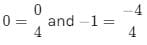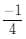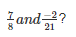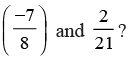Test: Rational Numbers

# Test: Rational Numbers

Test Description

## 10 Questions MCQ Test Class 8 Mathematics by VP Classes | Test: Rational Numbers

Test: Rational Numbers for Class 8 2022 is part of Class 8 Mathematics by VP Classes preparation. The Test: Rational Numbers questions and answers have been prepared according to the Class 8 exam syllabus.The Test: Rational Numbers MCQs are made for Class 8 2022 Exam. Find important definitions, questions, notes, meanings, examples, exercises, MCQs and online tests for Test: Rational Numbers below.
Solutions of Test: Rational Numbers questions in English are available as part of our Class 8 Mathematics by VP Classes for Class 8 & Test: Rational Numbers solutions in Hindi for Class 8 Mathematics by VP Classes course. Download more important topics, notes, lectures and mock test series for Class 8 Exam by signing up for free. Attempt Test: Rational Numbers | 10 questions in 20 minutes | Mock test for Class 8 preparation | Free important questions MCQ to study Class 8 Mathematics by VP Classes for Class 8 Exam | Download free PDF with solutions
 1 Crore+ students have signed up on EduRev. Have you?
Test: Rational Numbers - Question 1

### What should be added to -3/4 to get ‘–1’?

Test: Rational Numbers - Question 2

### What should be subtracted from −3/4 to get ‘–1’?

Test: Rational Numbers - Question 3

### Which of the following is the identity element of addition?

Detailed Solution for Test: Rational Numbers - Question 3

An identity element or neutral element is a special type of element of a set with respect to a binary operation on that set, which leaves any element of the set unchanged when combined with it

An identity with respect to addition is called an additive identity (often denoted as 0) .

Test: Rational Numbers - Question 4

Which of the following is the multiplicative identity for rational numbers?

Test: Rational Numbers - Question 5

Which of the following is neither positive nor a negative rational number?

Test: Rational Numbers - Question 6

Which of the following rational numbers lies between 0 and –1?

Detailed Solution for Test: Rational Numbers - Question 6

Clearly, 0 and −1 cannot lie between 0 and −1.
Also,We can clearly see thatlies between 0 and −1.

Test: Rational Numbers - Question 7

Which of the following is the reciprocal of ‘p’?

Test: Rational Numbers - Question 8

which of the following is the product of?

Test: Rational Numbers - Question 9

Which of the following is the product ofTest: Rational Numbers - Question 10

Which of the following is the reciprocal of the reciprocal of a rational number?

## Class 8 Mathematics by VP Classes

89 docs|16 tests
 Use Code STAYHOME200 and get INR 200 additional OFF Use Coupon Code
Information about Test: Rational Numbers Page
In this test you can find the Exam questions for Test: Rational Numbers solved & explained in the simplest way possible. Besides giving Questions and answers for Test: Rational Numbers , EduRev gives you an ample number of Online tests for practice

89 docs|16 tests